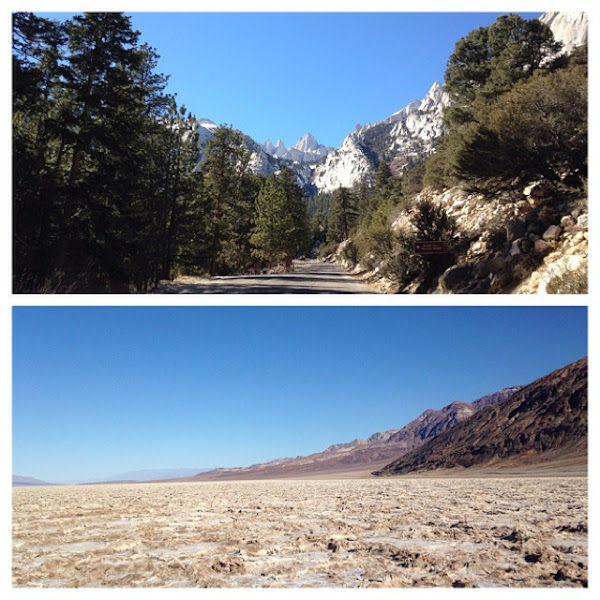## 20151121

### Physics quiz question: Mt. Whitney vs. Badwater Basin pressure difference

Physics 205A Quiz 5, fall semester 2015
Cuesta College, San Luis Obispo, CA"Ain't no mountain high enough!"
Christine
flic.kr/p/h9k5r4

The highest and lowest points[*] in the 48 contiguous states of the U.S are Mt. Whitney (4,421 m above sea level) and Badwater Basin (85 m below sea level). Assume that the variation of gravitational constant g and the density of air (ρair = 1.3 kg/m3) with elevation is negligible. The air pressure on top of Mt. Whitney is __________ less than the air pressure in Badwater Basin.
(A) 6.6×102 Pa.
(B) 4.5×104 Pa.
(C) 5.3×104 Pa.
(D) 1.024×105 Pa.

[*] wki.pe/Extreme_points_of_the_United_States.

Correct answer (highlight to unhide): (C)

For static fluids, the energy density relation between pressure difference ∆P and elevation change ∆y is given by:

0 = ∆P + ρ·g·∆y.

The difference in pressure ∆P between Mt. Whitney and Badwater Basin is then:

P = –ρ·g·∆y,

P = –(1.3 kg/m3)·(9.80 m/s2)·((+4,421 m) – (–85 m)),

P = –(1.3 kg/m3)·(9.80 m/s2)·(+4,506 m),

P = –57,406.44 Pa,

such that the difference in pressure between Mt. Whitney and Badwater Basin (to two significant figures) is 5.7×104 Pa (the negative sign in the above calculation denotes that the air pressure at Mt. Whitney is less than the air pressure in Badwater Basin).

(Response (A) is ρ·g·(yWhitney/yBadwater); response (B) is the absolute air pressure atop Mt. Whitney; and response (D) is the absolute air pressure in Badwater Basin.)

Sections 70854, 70855, 73320
Exam code: quiz05bL8D
(A) : 17 students
(B) : 0 students
(C) : 43 students
(D) : 5 students

Success level: 66%
Discrimination index (Aubrecht & Aubrecht, 1983): 0.63